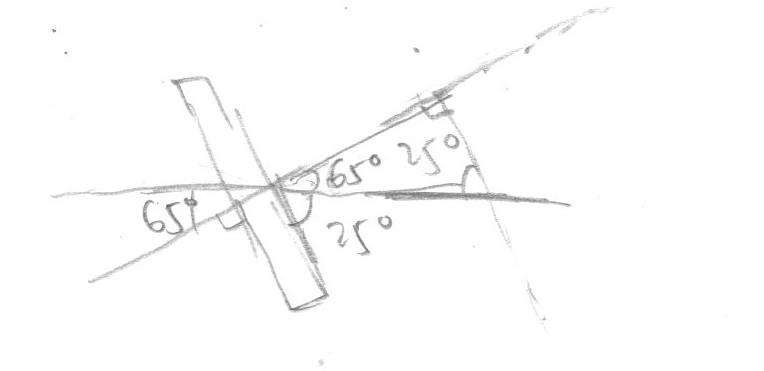# Force exerted normal to the plate

• foo9008
Check your arithmetic again.In summary, the conversation discusses the determination of the normal force exerted on a stationary flat plate when a jet of water with specific diameter and velocity strikes it at a 25 degree angle. The equation used to calculate the normal force is F = ρA(v)(v cos theta), and the resulting answer is 268.8N. The person asking for confirmation of their answer is advised to double check their arithmetic.

## Homework Statement

A jet of water , 50mm diameter , with a velocity of 18m/s , strikes a flat plate inclined at 25 degree to the axis of jet as shown in figure, Determine the normal force , P exerted on the plate when plate is stationary .

## The Attempt at a Solutionin the diagram attached , i found that the angle between the water velocity make an angle of 65 degree to the normal of the plate ,
so my ans is F = ρA(v)(v cos theta) = 1000(pi)( [(50x10^-3) / 2 ] ^2 ) (18^2)(cos 65) = 278.7 N , is my working correct ? i don't have the ans

Last edited:
foo9008 said:

## Homework Statement

A jet of water , 50mm diameter , with a velocity of 18m/s , strikes a flat plate inclined at 25 degree to the axis of jet as shown in figure, Determine the normal force , P exerted on the plate when plate is stationary .

## The Attempt at a Solutionin the diagram attached , i found that the angle between the water velocity make an angle of 65 degree to the normal of the plate ,
so my ans is F = ρA(v)(v cos theta) = 1000(pi)( [(50x10^-3) / 2 ] ^2 ) (18^2)(cos 65) = 278.7 N , is my working correct ? i don't have the ans